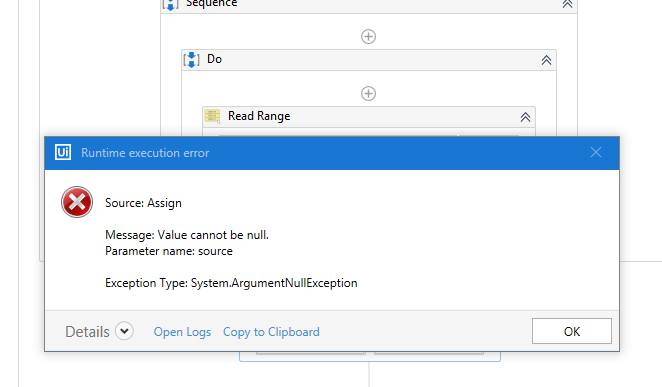# Calculate sum of excel column

Hi ,

I have an excel file containing Amount column. I need to calculate the sum of that column. How can I calculate sum of an excel column? Please suggest the solution.

Thanks,

Saili

@saili

Use Aggregation activity avaiable in Balreva.Datatable.Activities .

in this select aggregation method as sum and give the input as column name.

Regards,
A Manohar

1 Like

@saili,
Try the following inside an assign activity,

`````` yourDataTable.AsEnumerable().Sum(Function(r) r.Field(Of Integer)("yourColumnName"))
``````

For this you need to read your excel data as a datatable using Excel application scope

Got it. Thank You.

1 Like

Hi @sarathi125 ,

Good Day!

I am getting below error,what could be the reason:
Steps Followed:
Excel Application Scope with Read Range
Assign Activity:yourDataTable.AsEnumerable().Sum(Function(r) r.Field(Of Integer)(“yourColumnName”))
Write RangeyourDataTable - your excel data - datatable
yourColumnName - Are you passing correct column name.

Hi @sarathi125 ,

Thank you for the response,column name i have given correctly.
Could you please check my xaml.SumofExcelColumns.xaml (6.7 KB)

I have checked your xaml, if your column has very minimal value you can use Integer else if it has large values, then use Double as the following in your assign activity.

``````dtExcel.AsEnumerable().Sum(Function(r) r.Field(Of Double)("Amount"))
``````

And in the Write Range, which datatable you are referring. I can see you are holding the sum value in a result integer variable, but write dtResult which is empty will not get the expected result.

You need the result value in the new excel, then use write cell since the value is a single.

1 Like

Hi @sarathi125 ,

I have calculated the sum and pasted in excel with the changes you have done.
How to calculate the same for multiple columns for example same sheet contains columns:
Amount|Amount1|Amount2

If you looking for different column, then try with this,

``````Convert.ToInt32(dt.Compute("SUM(Amount)", string.Empty))
``````

Here you can change the Amount column to all the column names one by one.

You can also try

``````Create 3 variables with int32 datatype named as totalAmount1, totalAmount2, totalAmount3

totalAmount1 = (TryCast((From s In yourDataTable.AsEnumerable() Select Integer.Parse(s(1).ToString())), IEnumerable(Of Integer))).Sum()
totalAmount2 = (TryCast((From s In yourDataTable.AsEnumerable() Select Integer.Parse(s(2).ToString())), IEnumerable(Of Integer))).Sum()
totalAmount3 = (TryCast((From s In yourDataTable.AsEnumerable() Select Integer.Parse(s(3).ToString())), IEnumerable(Of Integer))).Sum()

``````

Here 1 - Amount1, 2-Amount2, 3-Amount3 column indexes.
This will add the total row in the same datatable at the bottom.

Good Morning!

I have tried the above code with first line of code and using message box printed total amount 1 but I am getting below error.
totalamount1:Int32(Variable)

If the Change variable type to Int64 or GenericValue still getting same error.

Check on which column you are getting this error, looks like some invalid data are there in the column.

As you said you are getting the correct output in a messagebox, then in which activity you are getting this error.

Hi @sarathi125 ,

I see some negative values are there in columns ,the error is causing because of that?

As you said you are getting the correct output in a messagebox, then in which activity you are getting this error(I just added this activity to check each column but not printed).

Then check all the columns values, and also while reading the negative values check how it is coming in the code, try to print those using writeline and check in the output window.

Possible share us a sample excel here.

Added the excel file here.Compare File_1.xlsx (1.1 MB)

It looks you have decimal values in that excel, Try with the below line of code.

``````(TryCast((From s In yourDataTable.AsEnumerable() Select Decimal.Parse(s(1).ToString())), IEnumerable(Of Decimal))).Sum()
``````

SQLUpdate.xaml (4.5 KB)
Check the attached xaml which will return sum of Amount1 column from sheet1.

For the first column(Amount) its working .second column still getting same error may be data issue.
Could you also give your inputs where we have to write the below code,Assign activity or Write cell?